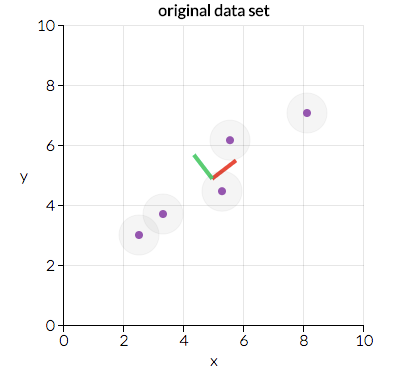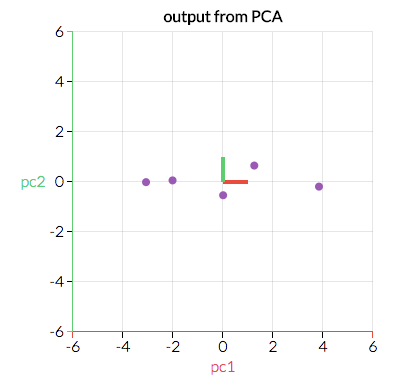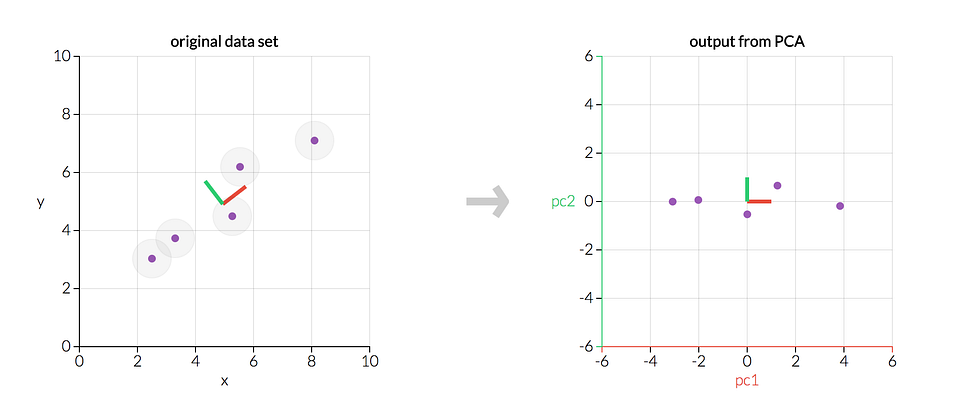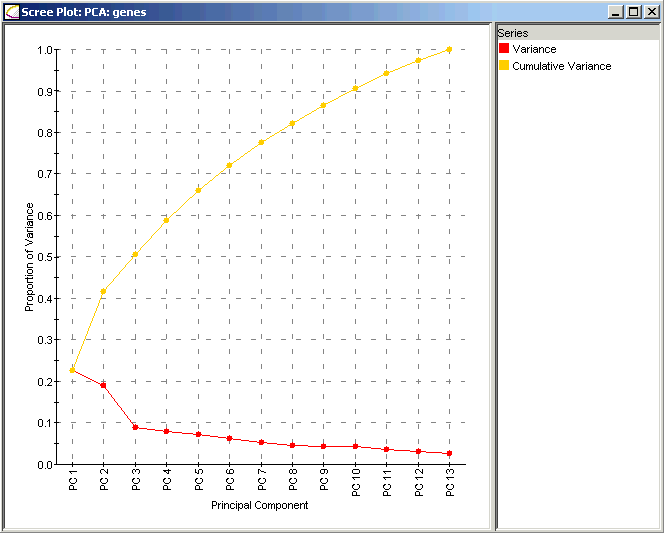2021-08-06
2021-08-22

## What is PCA?

Let’s say that you want to predict what the gross domestic product (GDP) of the United States will be for 2017. You have lots of information available: the U.S. GDP for the first quarter of 2017, the U.S. GDP for the entirety of 2016, 2015, and so on. You have any publicly-available economic indicator, like the unemployment rate, inflation rate, and so on. You have U.S. Census data from 2010 estimating how many Americans work in each industry and American Community Survey data updating those estimates in between each census. You know how many members of the House and Senate belong to each political party. You could gather stock price data, the number of IPOs occurring in a year, and how many CEOs seem to be mounting a bid for public office. Despite being an overwhelming number of variables to consider, this just scratches the surface.

you have a lot of variables to consider.

If you’ve worked with a lot of variables before, you know this can present problems. Do you understand the relationships between each variable? Do you have so many variables that you are in danger of overfitting your model to your data or that you might be violating assumptions of whichever modeling tactic you’re using?

You might ask the question, “How do I take all of the variables I’ve collected and focus on only a few of them?” In technical terms, you want to “reduce the dimension of your feature space.” By reducing the dimension of your feature space, you have fewer relationships between variables to consider and you are less likely to overfit your model. (Note: This doesn’t immediately mean that overfitting, etc. are no longer concerns — but we’re moving in the right direction!)

Somewhat unsurprisingly, reducing the dimension of the feature space is called “dimensionality reduction.” There are many ways to achieve dimensionality reduction, but most of these techniques fall into one of two classes:

• Feature Elimination
• Feature Extraction

Feature elimination is what it sounds like: we reduce the feature space by eliminating features. In the GDP example above, instead of considering every single variable, we might drop all variables except the three we think will best predict what the U.S.’s gross domestic product will look like. Advantages of feature elimination methods include simplicity and maintaining the interpretability of your variables.

As a disadvantage, though, you gain no information from those variables you’ve dropped. If we only use last year’s GDP, the proportion of the population in manufacturing jobs per the most recent American Community Survey numbers, and the unemployment rate to predict this year’s GDP, we’re missing out on whatever the dropped variables could contribute to our model. By eliminating features, we’ve also entirely eliminated any benefits those dropped variables would bring.

Feature extraction, however, doesn’t run into this problem. Say we have ten independent variables. In feature extraction, we create ten “new” independent variables, where each “new” independent variable is a combination of each of the ten “old” independent variables. However, we create these new independent variables in a specific way and order these new variables by how well they predict our dependent variable.

You might say, “Where does the dimensionality reduction come into play?” Well, we keep as many of the new independent variables as we want, but we drop the “least important ones.” Because we ordered the new variables by how well they predict our dependent variable, we know which variable is the most important and least important. But — and here’s the kicker — because these new independent variables are combinations of our old ones, we’re still keeping the most valuable parts of our old variables, even when we drop one or more of these “new” variables!

The principal component analysis is a technique for feature extraction — so it combines our input variables in a specific way, then we can drop the “least important” variables while still retaining the most valuable parts of all of the variables! As an added benefit, each of the “new” variables after PCA are all independent of one another. This is a benefit because the assumptions of a linear model require our independent variables to be independent of one another. If we decide to fit a linear regression model with these “new” variables (see “principal component regression” below), this assumption will necessarily be satisfied.

## When should I use PCA?

1. Do you want to reduce the number of variables, but aren’t able to identify variables to completely remove from consideration?
2. Do you want to ensure your variables are independent of one another?
3. Are you comfortable making your independent variables less interpretable?

If you answered “yes” to all three questions, then PCA is a good method to use. If you answered “no” to question 3, you should not use PCA.

## How does PCA work?

The section after this discusses why PCA works, but providing a brief summary before jumping into the algorithm may be helpful for context:

• We are going to calculate a matrix that summarizes how our variables all relate to one another.
• We’ll then break this matrix down into two separate components: direction and magnitude. We can then understand the “directions” of our data and its “magnitude” (or how “important” each direction is). The screenshot below, from the setosa.io applet, displays the two main directions in this data: the “red direction” and the “green direction.” In this case, the “red direction” is the more important one. We’ll get into why this is the case later, but given how the dots are arranged, can you see why the “red direction” looks more important than the “green direction?” (Hint: What would fitting a line of best fit to this data look like?)Our original data in the xy-plane. (Source.)
• We will transform our original data to align with these important directions (which are combinations of our original variables). The screenshot below (again from setosa.io) is the same exact data as above, but transformed so that the x– and y-axes are now the “red direction” and “green direction.” What would the line of best fit look like here?The original data transformed by PCA. (Source.)
• While the visual example here is two-dimensional (and thus we have two “directions”), think about a case where our data has more dimensions. By identifying which “directions” are most “important,” we can compress or project our data into a smaller space by dropping the “directions” that are the “least important.” By projecting our data into a smaller space, we’re reducing the dimensionality of our feature space… but because we’ve transformed our data in these different “directions,” we’ve made sure to keep all original variables in our model!

Here, I walk through an algorithm for conducting PCA. I try to avoid being too technical, but it’s impossible to ignore the details here, so my goal is to walk through things as explicitly as possible. A deeper intuition of why the algorithm works is presented in the next section.

Before starting, you should have tabular data organized with n rows and likely p+1 columns, where one column corresponds to your dependent variable (usually denoted Y) and p columns where each corresponds to an independent variable (the matrix of which is usually denoted X).

1. If a Y variable exists and is part of your data, then separate your data into Y and X, as defined above — we’ll mostly be working with X. (Note: if there exists no column for Y, that’s okay — skip to the next point!)
2. Take the matrix of independent variables X and, for each column, subtract the mean of that column from each entry. (This ensures that each column has a mean of zero.)
3. Decide whether or not to standardize. Given the columns of X, are features with higher variance more important than features with lower variance, or is the importance of features independent of the variance? (In this case, importance means how well that feature predicts Y.) If the importance of features is independent of the variance of the features, then divide each observation in a column by that column’s standard deviation. (This, combined with step 2, standardizes each column of X to make sure each column has a mean zero and standard deviation of 1.) Call the centered (and possibly standardized) matrix Z.
4. Take the matrix Ztranspose it, and multiply the transposed matrix by Z. (Writing this out mathematically, we would write this as ZZ.) The resulting matrix is the covariance matrix of Z, up to a constant.
5. Calculate the eigenvectors and their corresponding eigenvalues of ZZ. This is quite easily done in most computing packages— in fact, the eigendecomposition of ZZ is where we decompose ZZ into PDP⁻¹, where P is the matrix of eigenvectors and D is the diagonal matrix with eigenvalues on the diagonal and values of zero everywhere else. The eigenvalues on the diagonal of D will be associated with the corresponding column in P — that is, the first element of D is λ₁ and the corresponding eigenvector is the first column of P. This holds for all elements in D and their corresponding eigenvectors in P. We will always be able to calculate PDP⁻¹ in this fashion. (Bonus: for those interested, we can always calculate PDP⁻¹ in this fashion because ZZ is a symmetricpositive semidefinite matrix.)
6. Take the eigenvalues λ₁, λ₂, …, λp and sort them from largest to smallest. In doing so, sort the eigenvectors in P accordingly. (For example, if λ₂ is the largest eigenvalue, then take the second column of P and place it in the first column position.) Depending on the computing package, this may be done automatically. Call this sorted matrix of eigenvectors P*. (The columns of P* should be the same as the columns of P, but perhaps in a different order.) Note that these eigenvectors are independent of one another.
7. Calculate Z* = ZP*. This new matrix, Z*, is a centered/standardized version of X but now each observation is a combination of the original variables, where the weights are determined by the eigenvector. Because our eigenvectors in P* are independent of one another, each column of Z* is also independent of one another!An example from setosa.io where we transform five data points using PCA. The left graph is our original data X; the right graph would be our transformed data Z*.

Note two things in this graphic:

• The two charts show the exact same data, but the right graph reflects the original data transformed so that our axes are now the principal components.
• In both graphs, the principal components are perpendicular to one another. In fact, every principal component will ALWAYS be orthogonal (a.k.a. official math term for perpendicular) to every other principal component. (Try to break the applet!)

Because our principal components are orthogonal to one another, they are statistically linearly independent of one another… which is why our columns of Z* are linearly independent of one another!

## How to determine the number of Principal Components to keep?

Finally, we need to determine how many features to keep versus how many to drop. There are three common methods to determine this, discussed below and followed by an explicit example:

• Method 1: We arbitrarily select how many dimensions we want to keep. Perhaps I want to visually represent things in two dimensions, so I may only keep two features. This is use-case dependent and there isn’t a hard-and-fast rule for how many features I should pick.
• Method 2: Calculate the proportion of variance explained (briefly explained below) for each feature, pick a threshold, and add features until you hit that threshold. (For example, if you want to explain 80% of the total variability possibly explained by your model, add features with the largest explained proportion of variance until your proportion of variance explained hits or exceeds 80%.)
• Method 3: This is closely related to Method 2. Calculate the proportion of variance explained for each feature, sort features by the proportion of variance explained, and plot the cumulative proportion of variance explained as you keep more features. This plot is called a scree plot, shown below. One can pick how many features to include by identifying the point where adding a new feature has a significant drop in variance explained relative to the previous feature, and choosing features up until that point. (I call this the “find the elbow” method, as looking at the “bend” or “elbow” in the scree plot determines where the biggest drop in the proportion of variance explained occurs.)

Because each eigenvalue is roughly the importance of its corresponding eigenvector, the proportion of variance explained is the sum of the eigenvalues of the features you kept divided by the sum of the eigenvalues of all features.Scree Plot for Genetic Data. (Source.)

Consider this scree plot for genetic data. (Source: here.) The red line indicates the proportion of variance explained by each feature, which is calculated by taking that principal component’s eigenvalue divided by the sum of all eigenvalues. The proportion of variance explained by including only principal component 1 is λ₁/(λ₁ + λ₂ + … + λp), which is about 23%. The proportion of variance explained by including only principal component 2 is λ₂/(λ₁ + λ₂ + … + λp), or about 19%.

The proportion of variance explained by including both principal components 1 and 2 is (λ₁ + λ₂)/(λ₁ + λ₂ + … + λp), which is about 42%. This is where the yellow line comes in; the yellow line indicates the cumulative proportion of variance explained if you included all principal components up to that point. For example, the yellow dot above PC2 indicates that including principal components 1 and 2 will explain about 42% of the total variance in the model.

Now let’s go through some examples:

• Method 1: We arbitrarily select a number of principal components to include. Suppose I wanted to keep five principal components in my model. In the genetic data case above, these five principal components explain about 66% of the total variability that would be explained by including all 13 principal components.
• Method 2: Suppose I wanted to include enough principal components to explain 90% of the total variability explained by all 13 principal components. In the genetic data case above, I would include the first 10 principal components and drop the final three variables from Z*.
• Method 3: Here, we want to “find the elbow.” In the scree plot above, we see there’s a big drop in the proportion of variability explained between principal component 2 and principal component 3. In this case, we’d likely include the first two features and drop the remaining features. As you can see, this method is a bit subjective as “elbow” doesn’t have a mathematically precise definition and, in this case, we’d include a model that explains only about 42% of the total variability.

(Note: Some scree plots will have the size of eigenvectors on the Y axis rather than the proportion of variance. This leads to equivalent results but requires the user to manually calculate the proportion of variance. An example of this can be seen here.)

Once we’ve dropped the transformed variables we want to drop, we’re done! That’s PCA.

## Why does PCA work?

While PCA is a very technical method relying on in-depth linear algebra algorithms, it’s a relatively intuitive method when you think about it.

• First, the covariance matrix ZZ is a matrix that contains estimates of how every variable in Z relates to every other variable in Z. Understanding how one variable is associated with another is quite powerful.
• Second, eigenvalues and eigenvectors are important. Eigenvectors represent directions. Think of plotting your data on a multidimensional scatterplot. Then one can think of an individual eigenvector as a particular “direction” in your scatterplot of data. Eigenvalues represent magnitude or importance. Bigger eigenvalues correlate with more important directions.
• Finally, we make an assumption that more variability in a particular direction correlates with explaining the behavior of the dependent variable. High variability usually indicates signal, whereas little variability usually indicates noise. Thus, the more variability there is in a particular direction is, theoretically, indicative of something important we want to detect. (The setosa.io PCA applet is a great way to play around with data and convince yourself why it makes sense.)

Thus, PCA is a method that brings together:

1. A measure of how each variable is associated with one another. (Covariance matrix.)
2. The directions in which our data are dispersed. (Eigenvectors.)
3. The relative importance of these different directions. (Eigenvalues.)

PCA combines our predictors and allows us to drop the eigenvectors that are relatively unimportant.

Reference:

https://towardsdatascience.com/a-one-stop-shop-for-principal-component-analysis-5582fb7e0a9c

https://ourarchive.otago.ac.nz/bitstream/handle/10523/7534/OUCS-2002-12.pdf?sequence=1&isAllowed=y##### Amir Masoud Sefidian
Machine Learning Engineer